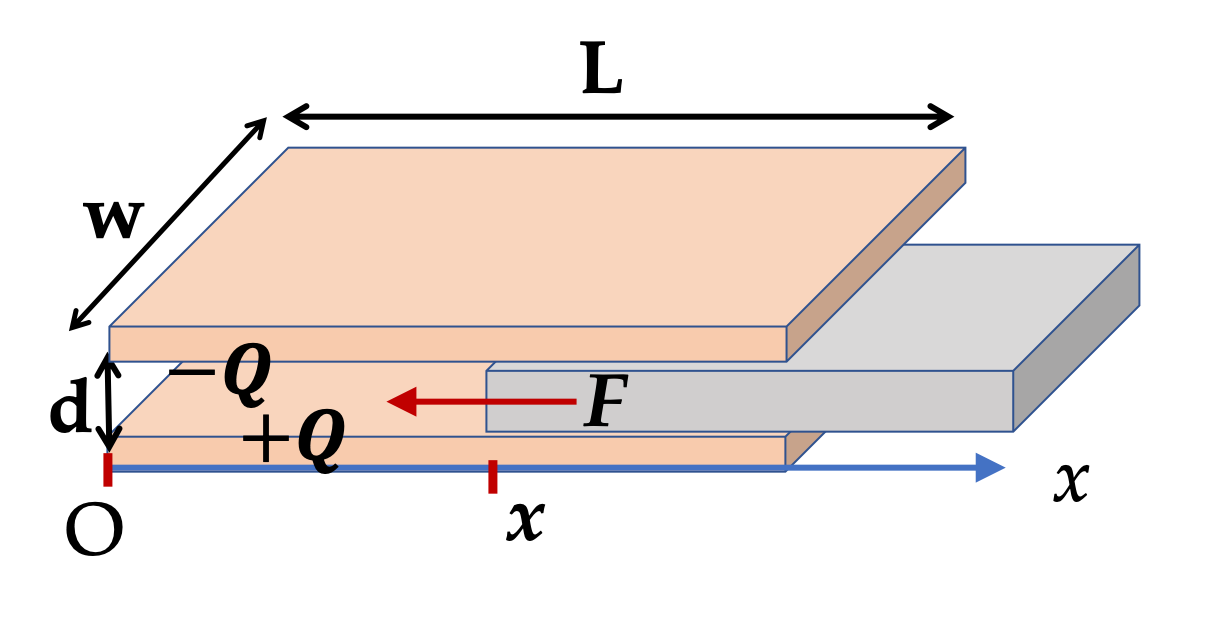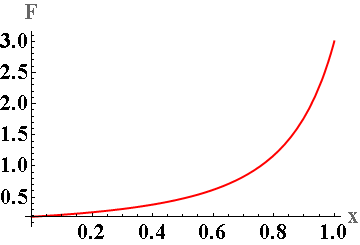## Section33.6Forces on Dielectrics

When you place a dielectric in a uniform electric field, the net force on the dielectric must be zero because the sum of forces on each dipole is zero. But, if you put a dielectric in a non-uniform electric field, there will be a net force on each dipole due to different electric field at the positive charge than on the negative charge. As a result, the dielectric will be pulled towards region of increasing electric field.

For instance, if you place a dielectric at the edge of parallel plate, which have been charge by opposite chrges, the non-uniformity of the field at the edge will tend to pull the dielectric.

Suppose $\pm Q$ are on the plates separated by a distance $d\text{.}$ Let $w$ be the widths of the plate and $L$ the length, and at some instant, let $L-x$ along the length have a dielectric of dielectric constant $\epsilon_r$ and length $x$ be empty.We will show below that the dielectric slab is pulled into the slab by the following force be in the negative $x$ direction, given by

\begin{equation*} F_x = - \frac{1}{2}\ \frac{Q^2d}{\epsilon_0 w}\ \frac{\epsilon_r - 1}{\left[ x + \epsilon_r(L-x)\right]^2}. \end{equation*}

This is a complicated formula, which we will prove in the chapter on capacitors. The following plot shows that when the entire dielectric is just outside the edge, force is greates, and as the dielectric gets pulled in further and further, the force decreases to zero when the dielectric is all the way in, which is at $x=0$ in the plot.Figure 33.6.1. Force on a dielectric as the dielectric gets pulled in. As the dielectric enters the space between the plots, near $x=L\text{,}$ the force is strongest. The force, gradually and non-linearly, decreases to zero at the instant the dielectric is fully in as indicated at $x=0\text{.}$ I have set $\frac{Q^2d}{\epsilon_0 w} = 2\text{,}$ $\epsilon_r=4\text{,}$ and $L=1\text{.}$